# Photometric Stereo

### Chaman Singh Verma and Mon-Ju Wu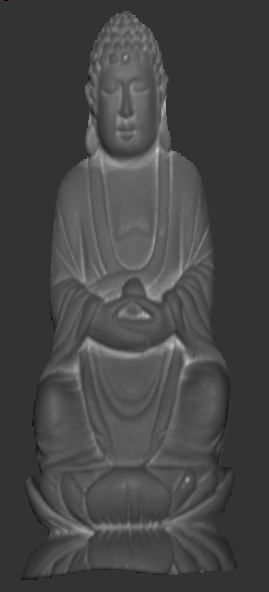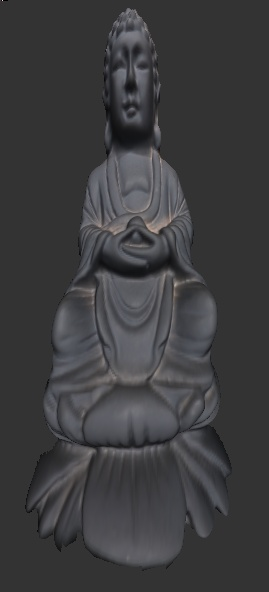Original Image Albedo Normal Map Normal Vectors Height Map 3D Rendering

### Introduction:

Photometric stereo is a technique to estimate depth and surface orientation from images of the same view taken from different directions. Theoretically, only three directions are sufficient to obtain normals, but to minimize noises inherent in the process, more than minimum number is often required for realistic images. This method, however has some limitations (1) Light source must be far from the objects (2) Specular or dark spots in the regions don't give satisfactory results (3) shadows must be masked to avoid for valid 3D surface reconstruction.

There are two coordinate systems (1) Image based coordinate system in which upper lower is the origin and downward "y" is positive (2) Right hand world coordinate system in which x goes from left to right, y from bottom to top and "z" from back to front.  Throughout the project,  we shall stick to right hand world coordinate system.

### Light Calibration

The first step in the calculations of normal map is to calibrate light source i.e estimate the light direction. One way to do this is to use chrome ball on which the brightest spot is used to  identify the direction of the light.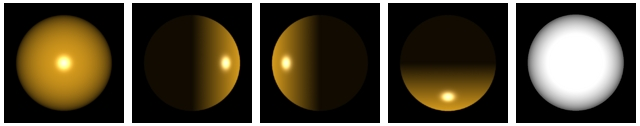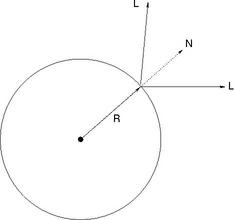Synthetic data generated using OpenGL to verify light caliberation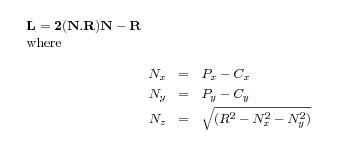Where R is the reflection direction taken as [0,0,1]. Here [Px,Py] is the location of brighest point on the chrome image and [Cx,Cy] is the center of the chrome in image space  which can be estimated by the maskimage/

### Normal Map Generation :

For lambertain surfaces, intentity at any point on the surface can be given as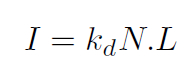Where N is the normal at the surface point and L is the reflected light direction. In order to determine N, we need at least three light source which are not in the same plane. Therefore the above equations can be written as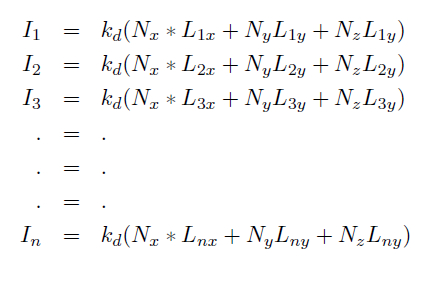Now let us denote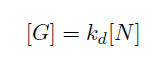So for n > 3, we can solve the equations with least square methods as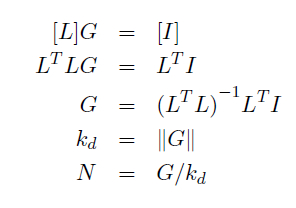Therefore, we can retrieve both normal and albedo map per pixel, if the pixel receives light from at least one source. In practice, there are many pixels in the region where light intensity from the sources is very low, so we need to skips those pixels for calculations. Matlab implementation of the this code is very straightforward.

### Depth Map Generation:The Normal at surface must be orthogonal to the vector V1 gives: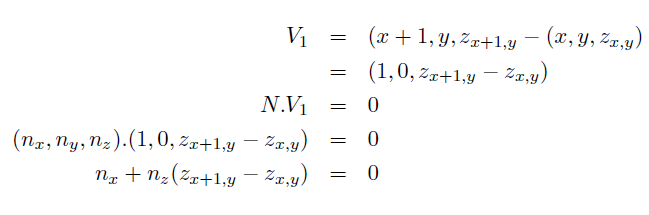The normal at surface must be orthogonal to the vector V2 gives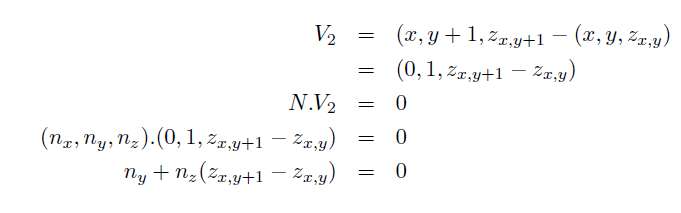Boundary conditions or pixels where either (nx,ny,nz) values are not available must be taken into consideration. At those pixels, instead of taking forward step for depth calculation we take forward direction that modifies the above equations as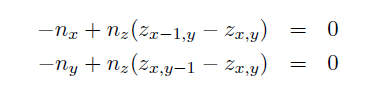This generate a sparse matrix of (2*numPixels, numPixels) ( where numPixels are the pixels in the masked region and not the entire region) where each row contains only two entries. This overdetermined system of liner equations is solved by least square method of conjugate gradient method by making equation symmetric and positive definate.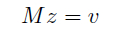or equivalent system: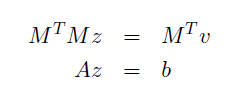## Results:

The following dataset were provided by Dr. Li Zhang for this class project.
• ### Dataset: Buddha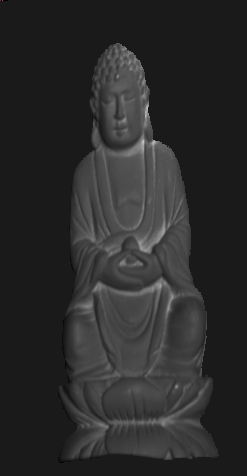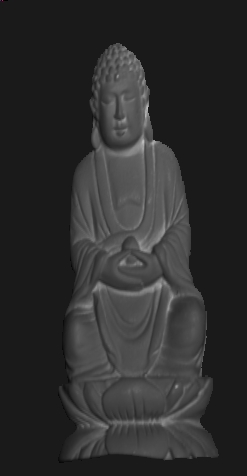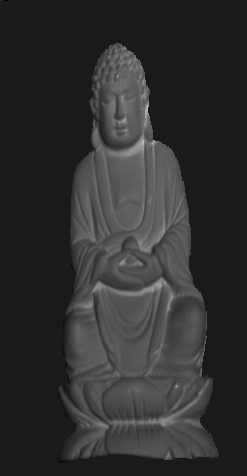Red Albedo Green Albedo Blue Albedo• ### Dataset: Cat

•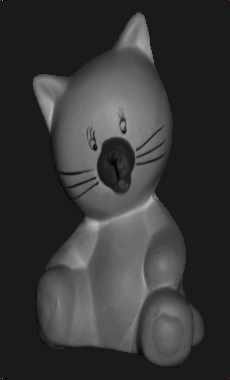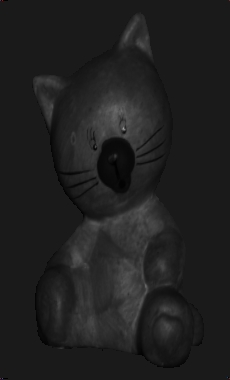Red Albedo Green Albedo Blue Albedo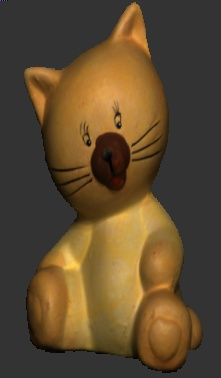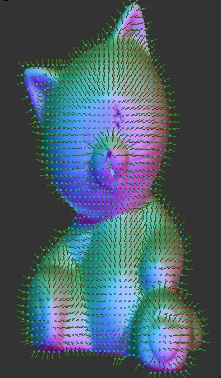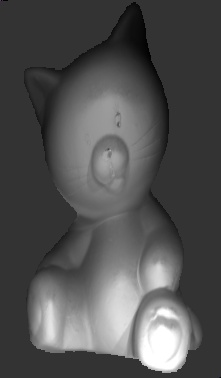Original Image Normal Map Normal Vectors HeightMap 3D Rendering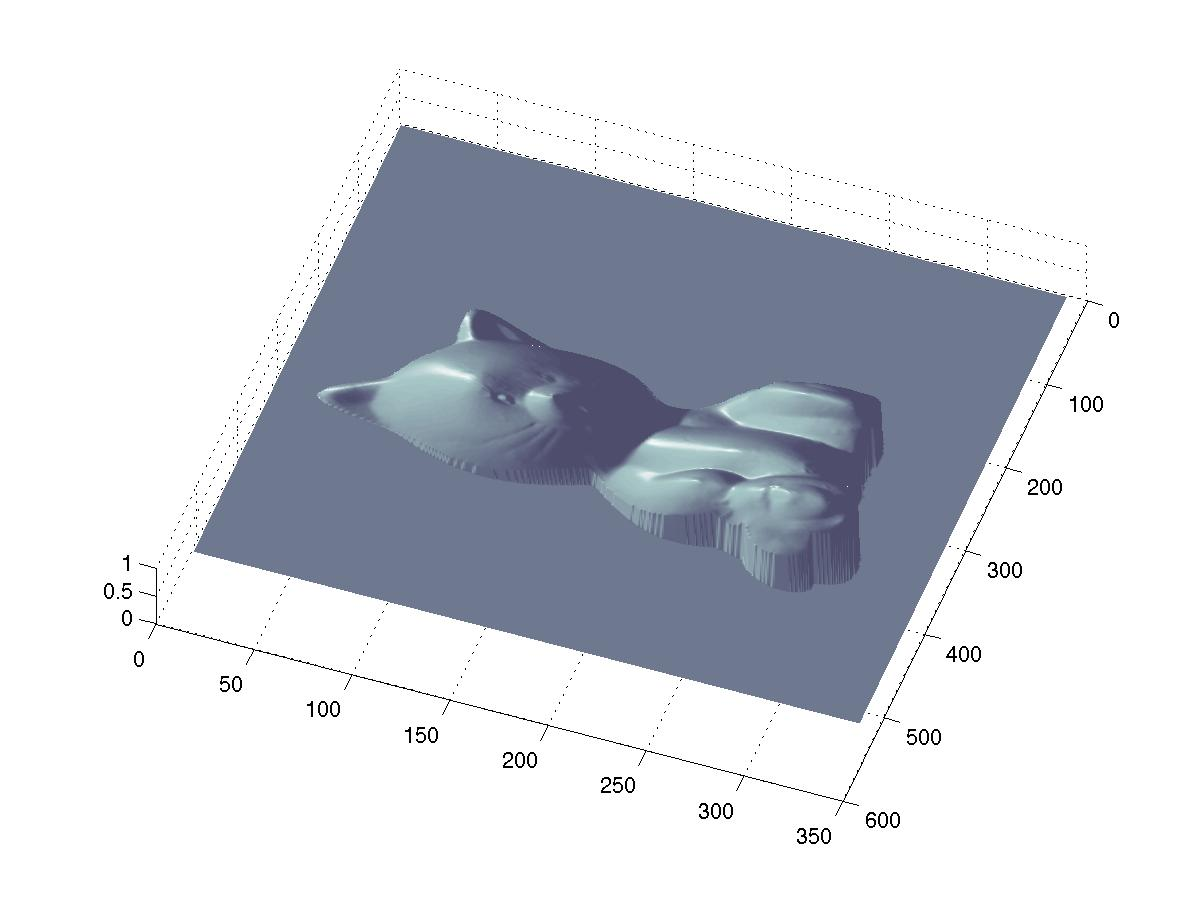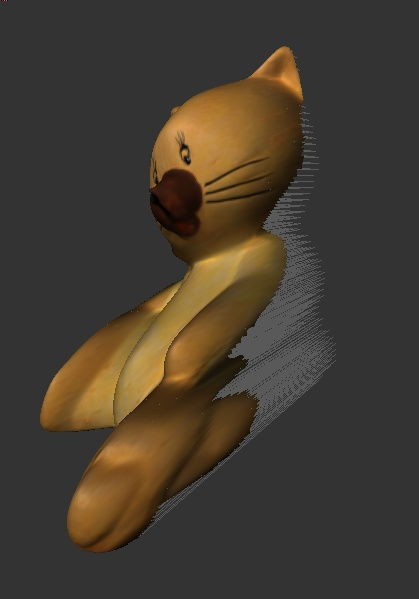• ### Dataset: Horse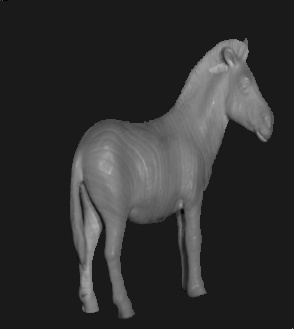Red Albedo Green Albedo Blue Albedo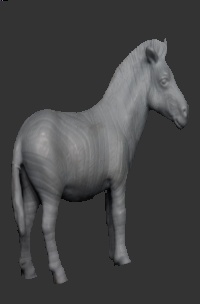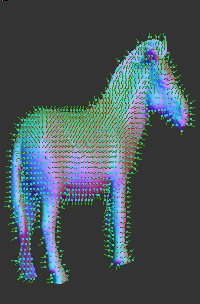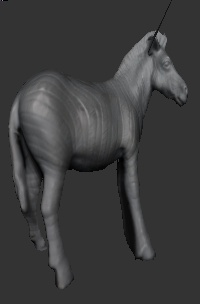Original Normal field Normal Vectors Height Map 3D Rendering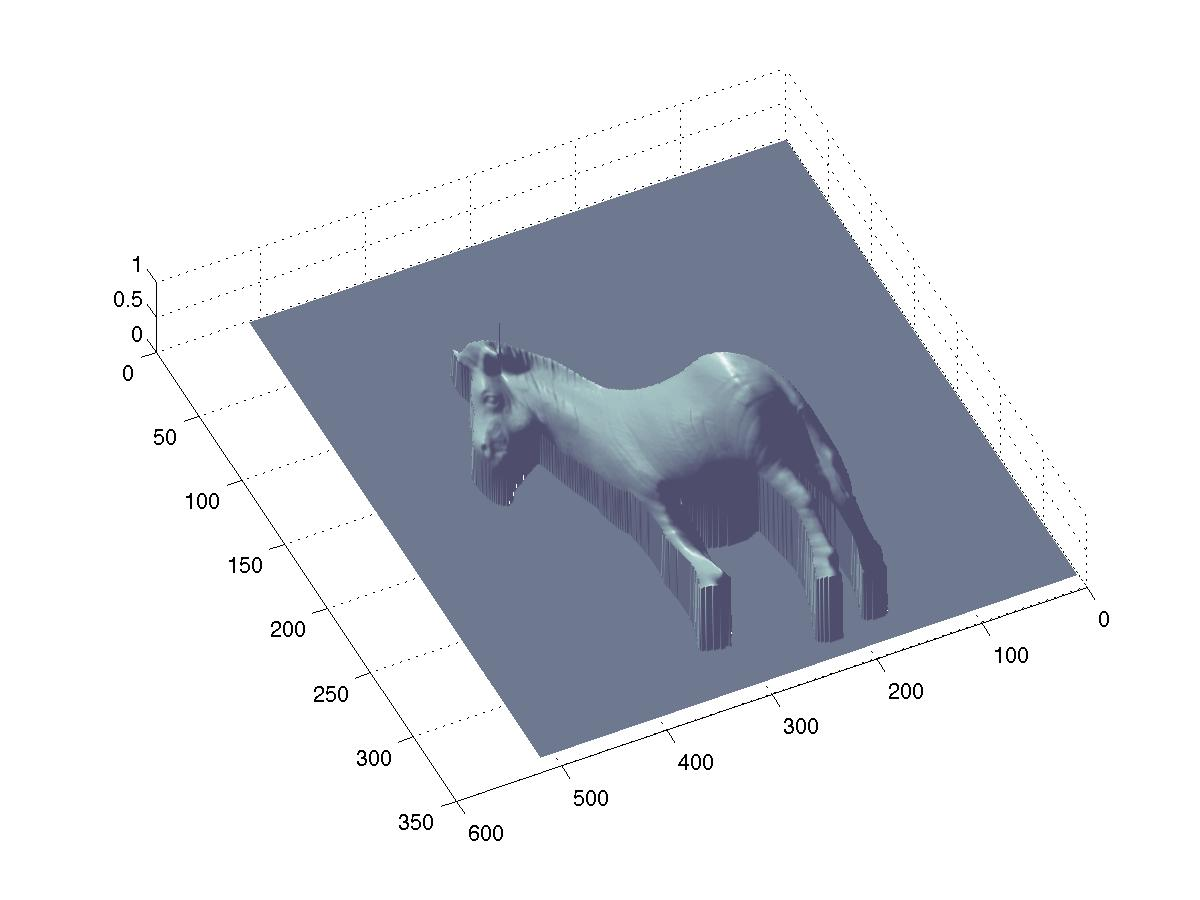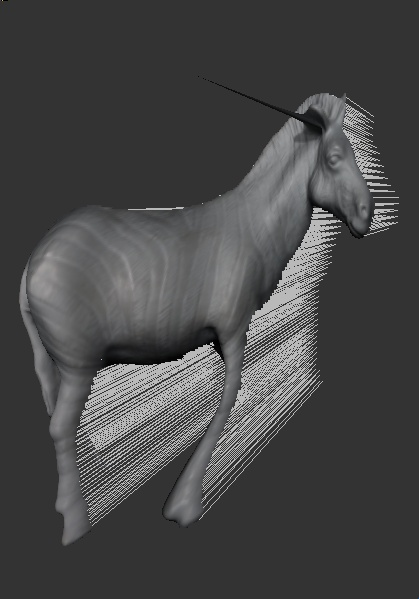• ### Dataset:Owl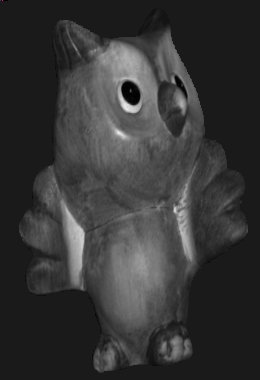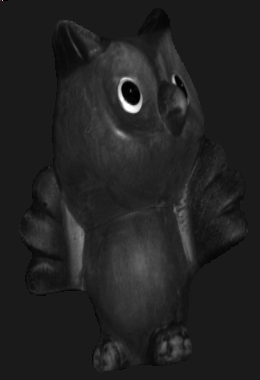Red Albedo Green Albedo Blue Albedo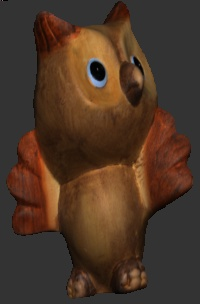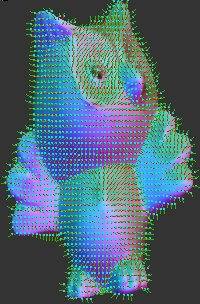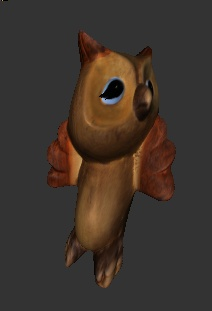Original Normal map Normal vectors Height Map 3D Rendering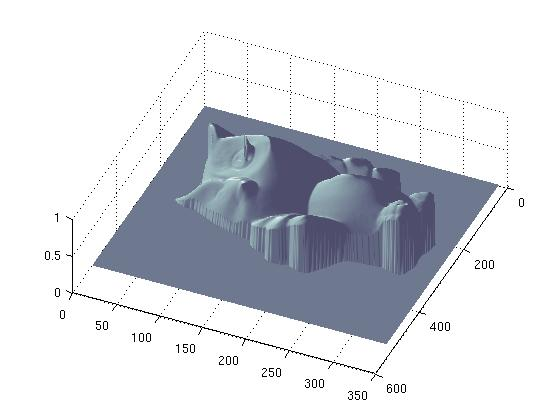• ### Dataset:Rock

•Red Albedo Green Albedo Blue Albedo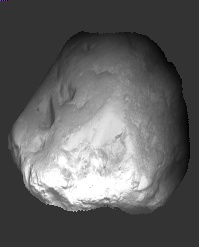Original Normal Map Normal Vectors Height Map 3D Rendering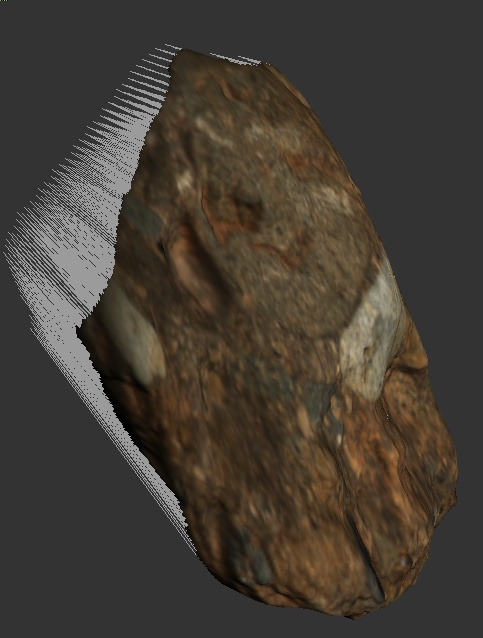### Discussions and Conclusions

1. It seems that present method (which is quite naive) is poor in handling dark spots in the image. Both owl's eyes and horse ears we can observe the spiked artifacts.
2. Although the owl's depth map looks quite reasonable, the 3D rendering is not impressive. There is definately distortion because of scaling effect.
3. Since the depth map calculations are valid for arbitrary scaling, the "Z' values are also scaled. Perhaps the Z scaling could be calculated by matching normals from the 3D reconstructed model. ( But we didn't carry out this exercise in this study).

### Software and User's Guide

The entire software is written in Matlab because it requires some sparse linear system solvers and it was quick to implement it. There are four main modules in this software
1. Stereo.m                :  Main Driver routine
2. CalibrateLight.m   :   Function to calibrate light with the given chrome ball.
3. NormalMap.m       :  Generate Normal Map given Light Directions
4. DepthMap.m         :  Generate Depth Map given Normal Map
The use of the software in simple, just write on Matlab command prompt

z = Stereo( directory, dataset, numImages)

Where "directory" folder contains  (1) A folder named dataset which contains all the images (2) "chrome" directory containing chrome ball information, which is used to  caliberating light directions.
The  third argument "numImages" is the number of images in the dataset directory.  Example

z = Stereo( './DataSet', 'buddha', 12 );

The image are generated using libQglViewer  library which is based on OpenGL and Qt. The source code of viewer is ( not user friendly right now ). main.cpp simpleViewer.h simpleViewer.cpp

### Group Work Contribution

Initially Mon-Ju did the light caliberation part and myself ( Chaman Singh ) developed the NormalMap.m and DepthMap.m codes in Matlab. But we faced lots of troubles in getting the right results. So after we decided that Mon-Ju should write a new Matlab with a fresh outlook and not taking any feedback from our previously developed code, so that we could debug and understand some missing points. We took lots of help from Joel, Adrian and Dr. Zhang for many issues in developing the final code. Although the entire project looked very simple in the beginning, it tooks lots of time in figuring out the problems and all of them were related to mixing coordinate systems from the paper, our own interpretation and openGL coordinate system. Perhaps the biggest lesson, we learned in this project is to choose one coordinate system and "remain consistent" with it thoughout. Although the crux of the code ( DepthMap.m) was written by Mon-Ju, it was significantly modularised and cleaned by me. All the final results, visualization and document were contributed by me.